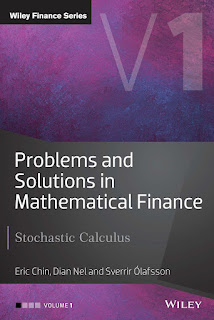CALCULUS MATHEMATICS

# Problems and Solutions in Mathematical Finance Volume1 Stochastic Calculus Free DownloadMathematical finance requires the use of advanced mathematical techniques drawn from the theory of probability, stochastic processes and stochastic differential equations. These areas are generally introduced and developed at an abstract level, making it problematic when applying these techniques to practical issues in finance. Problems and Solutions in Mathematical Finance Volume I: Stochastic Calculus is the first of a four-volume set of books focusing on problems and solutions in mathematical finance. This volume introduces the reader to the basic stochastic calculus concepts required for the study of this important subject, providing a large number of worked examples which enable the reader to build the necessary foundation for more practical orientated problems in the later volumes. Through this application and by working through the numerous examples, the reader will properly understand and appreciate the fundamentals that underpin mathematical finance. Written mainly for students, industry practitioners and those involved in teaching in this field of study, Stochastic Calculus provides a valuable reference book to complement one’s further understanding of mathematical finance.